# Laser Diode to Fiber

This article discusses how to use FRED to accurately model the coupling from a ball-lens capped semiconductor laser diode to a single mode fiber, an optical system common in optical fiber communication applications.

This model demonstrates FRED’s capability to propagate coherent fields, its accurate Laser Diode Beam source type, and its ability to calculate fiber coupling efficiency.

The semiconductor laser diode used in this FRED model is the Mitsubishi ML725C8F operating at 1310nm wavelength. The Mitsubishi source specifications define the output beam divergence angles in x and y to be 25 and 30 degrees respectively (full 1/e width of the power far field profile). No mention is made of any offsets in the focal positions in x and y, so they are assumed to be coincident and at the source.

This laser diode source is modeled in FRED using the Laser Diode Beam source type and is set to produce coherent input.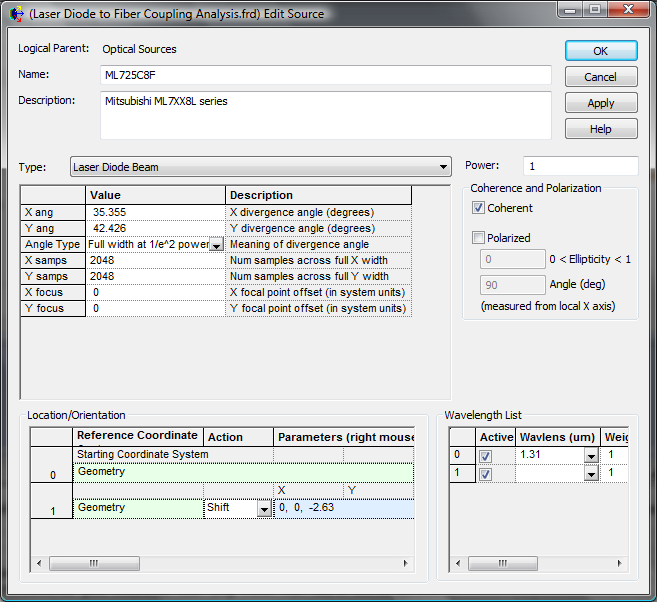In the settings for the Laser Diode Beam source, we have chosen to define the divergence angles using the 1/e2 power. This requires that the angles provided by the manufacturer are multiplied by a factor of sqrt(2).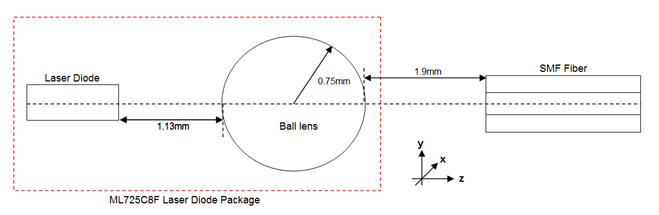The 1.5mm diameter ball lens is part of the Mitsubishi laser diode package and is positioned 1.88mm from the emitting surface of the laser diode. This is created in FRED using a spherical Element Primitive, and for convenience, the global origin is chosen to be where the output surface of the ball lens intercepts the optical axis.

The single mode fiber (SMF) used in the model is located 1.9mm from the global origin and its structure (defined in the figure below) is based on values that are typical for a SMF. The fiber core has radius 5 microns and is surrounded by a cladding that is 125 microns across. The refractive index values for the core and the cladding are 1.465 and 1.4. An absorbing coating, or jacket, that surrounds the fiber is also included in the model.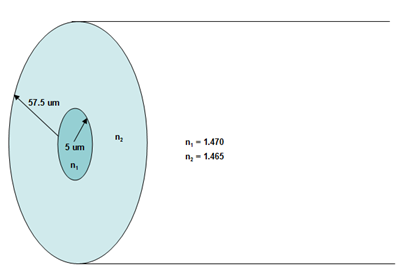The fiber is defined in FRED as a Subassembly consisting of multiple Element Primitives – a cylinder for the core, and pipes for the cladding and coating: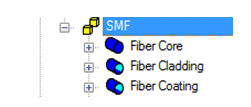The inside wall of the “Fiber Cladding” pipe exactly coincides with the outer wall of the “Fiber Core” cylinder. To model this correctly, we must manually set the Inner Wall of the cladding pipe to be Never Traceable. Failure to do this will lead to raytrace errors, as we have two surfaces located at exactly the same position in space and with two different sets of materials. The same needs to be done for the Inner Wall of “Fiber Coating.”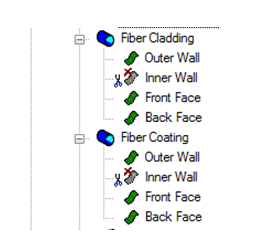In this model, the fiber coating is considered to be absorbing and has Halt All raytrace control. All other surfaces are uncoated.

FRED calculates the fiber coupling efficiency (CE) using the following equation: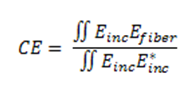where E inc is the incident field, and Efiber is the field profile of the fundamental mode of the fiber (calculated automatically by FRED given the fiber specifications).

In general, CE is a complex quantity, and therefore the coupled power is actually

CEpower = Re[CE]2 + Im[CE]2

Therefore, to calculate the fiber coupling accurately, an Analysis Surface needs to be located just behind the fiber entrance to ensure that the reflection coefficient at this interface is correctly taken into account.

It is important that the Analysis Surface is larger than the expected mode field diameter (MFD) of the fundamental mode so as to perform an accurate overlap integral. It is also important to be aware that the accuracy of this numerical integration is dependent on the number of divisions in the Analysis Surface. In this case, 251 x 251 on a 50um wide Analysis Surface is deemed sufficient.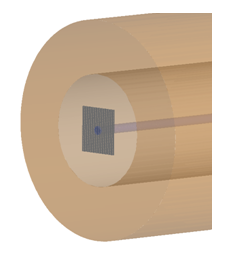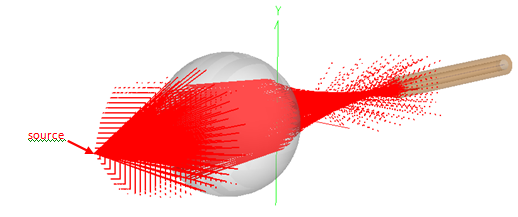The value returned by FRED’s fiber coupling efficiency calculation is the overlap fraction between the two field profiles. It does not take into account the power of the incident field. Therefore, understanding how much power is coupled to the mode must be done in two steps:

1. Determine the amount of power (P) at the Analysis Surface by performing an Irradiance calculation.
2. Determine the CE fraction from the fiber coupling efficiency analysis.

The amount of power that is coupled to the fiber mode is given simply by P * CE power.

After the raytrace, the fraction of source power that reaches the Analysis Surface behind the fiber interface is displayed in the Output Window when we calculate the Irradiance.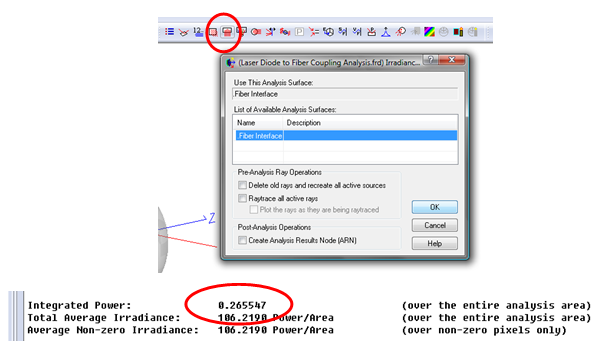26.55% of the source power reaches the Analysis Surface. FRED’s Fiber Coupling Efficiency analysis is used to determine the coupling to the fiber mode.  Note that the fiber core radius of 0.005mm has to be entered explicitly here.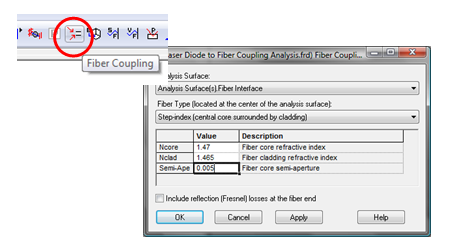Click on the OK button to display the results in the Output Window.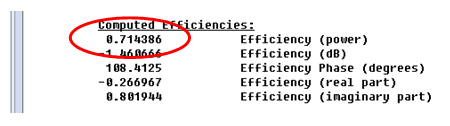The coupling efficiency is 71.44%. Therefore,  in this system the total coupled power percentage is 71.44% * 26.55% = 19.0%. The ML725C8F laser diode source operates at 5mW, which means that the fiber will transmit a signal of just under 1mW.

Associated FRED file:  Laser Diode to Fiber Coupling Analysis.frd

The above file includes three Embedded Scripts that study longitudinal, horizontal and angular alignment sensitivity of the fiber position and are discussed in our Outputting Data to Excel article.